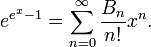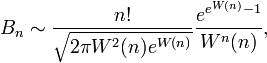# Partition (mathematics)

In mathematics, partition refers to two related concepts, in set theory and number theory.

## Partition (set theory)

A partition of a set X is a collection$\mathcal{P}$ of non-empty subsets ("parts") of X such that every element of X is in exactly one of the subsets in$\mathcal{P}$.

Hence a three-element set {a,b,c} has 5 partitions:

• {a,b,c}
• {a,b}, {c}
• {a,c}, {b}
• {b,c}, {a}
• {a}, {b}, {c}

Partitions and equivalence relations give the same data: the equivalence classes of an equivalence relation on a set X form a partition of the set X, and a partition$\mathcal{P}$ gives rise to an equivalence relation where two elements are equivalent if they are in the same part from$\mathcal{P}$.

The number of partitions of a finite set of size n into k parts is given by a Stirling number of the second kind;

### Bell numbers

The total number of partitions of a set of size n is given by the n-th Bell number, denoted Bn. These may be obtained by the recurrence relation$B_n=\sum_{k=0}^{n-1} \binom{m-1}{k} B_k .$

They have an exponential generating function$e^{e^x-1} = \sum_{n=0}^\infty \frac{B_n}{n!} x^n .$

Asymptotically,$B_n \sim \frac{n!}{\sqrt{2\pi W^2(n)e^{W(n)}}}\frac{e^{e^{W(n)}-1}}{W^n(n)},$

where W denotes the Lambert W function.

## Partition (number theory)

A partition of an integer n is an expression of n as a sum of positive integers ("parts"), with the order of the terms in the sum being disregarded.

Hence the number 3 has 3 partitions:

• 3
• 2+1
• 1+1+1

The number of partitions of n is given by the partition function p(n).